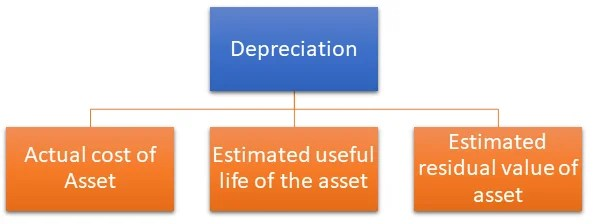# Factors affecting depreciation

26th November 2020
1. Normal Physical Wear and Tear:

Due to normal use of the assets, the assets deteriorate physically, which results in reduction in their value.

1. Efflux of Time:

Certain intangible assets have fixed life span such as Trade Marks, Patents or Copyrights etc. The value of such assets decreases anyway with the passage of time irrespective of the fact business enterprise is using them or not.

1. Obsolescence:

Research & Development leads to innovations, in the form of better and technically advanced machines that scrap old machines even though they may be capable of being run physically.

In that case there may be a permanent decrease in the market prices of certain assets like Computers, Motor Cars etc. This results in decline in the value of old machines. Obsolescence is a loss arising from outdating and replacing the existing asset with the new and improved model of that asset.

1. Accidents:

Destruction or damage caused by an accident may result in reducing the value of assets.

#### Factors Affecting Depreciation:

As already stated, depreciation is not an attempt to record the changes in the market value of the asset but a systematic allocation of the total cost of depreciable asset (capital expenditure) to expenses (revenue expenditure) over the useful life of the asset because market value of some assets may increase in short run but even then the depreciation process continues. Based on the matching principle a reasonable portion of capital expenditure (i.e. the cost of the asset) should be charged to revenue during the useful life of an asset.

The calculation of amount of depreciation expense for an accounting period is affected by the:(i) Actual cost of the asset

(ii) Estimated useful life of the asset

(iii) Estimated residual value of the asset.

It is worth mentioning here that out of three factors, two factors are based on just estimation and only one factor is based on actual. Thus, calculation of depreciation expense is just an estimated loss in value of assets and not the real and exact decrease in value of an asset.

Now we shall move on to discuss each of the above factors in detail:

1. Actual Cost of the Asset:

Actual cost or historical cost means the acquisition cost of the asset and includes all incidental expenses which are necessary to bring the asset to its present condition and location. Examples of such expenses are installation charges, freight inwards or expenses incurred for improvements of such assets and which are of capital nature.

1. Estimated Useful Life of the Asset:

Estimated useful life of the asset is either:

(i) The period over which a depreciable asset is expected to be used by the enterprise or

(ii) The number of production or similar units expected to be obtained from the use of the asset by the enterprise.

1. Estimated Residual or Scrap Value of the Asset:

Residual or scrap value is the expected value which may be realized when the asset is sold or exchanged at the end of its estimated useful life. When residual value is significant, it should be taken into consideration for computing depreciation. However, an insignificant residual value can be ignored for computation of depreciation.

Depreciation is a continuous process, but we don’t record depreciation daily. Actually, the total amount of depreciation to be charged on any asset is an advance expenditure which has been paid by the enterprise at the time of acquisition of such asset.

In other words, this expenditure should be treated like deferred expenditure and only adjusting entries, for charging reasonable and appropriate amount of depreciation to revenue in the income statement, are required to be passed every year.